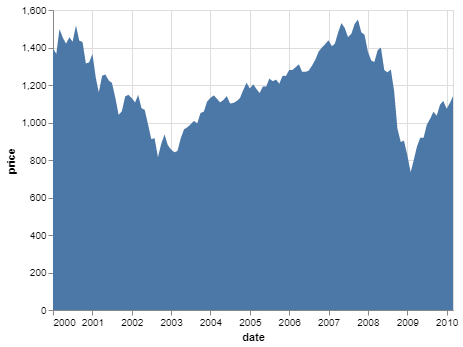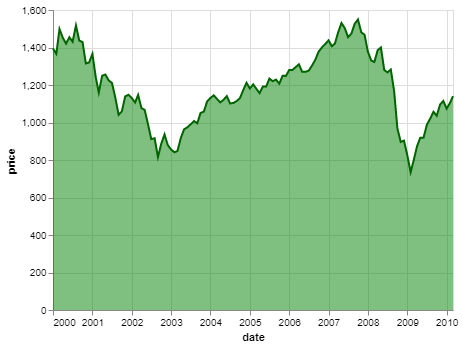Open in App
Not now

# Area Chart with Altair in Python

• Last Updated : 29 Sep, 2021

Prerequisite: Introduction to Altair in Python

An Area Graph shows the change in a quantitative quantity with respect to some other variable. It is simply a line chart where the area under the curve is colored/shaded. It is best used to visualize trends over a period of time, where you want to see how the value of one variable changes over a period of time or with respect to another variable and do not care about the exact data values. Some modifications of the area chart are the stacked area chart and streamgraph.

Area Graph is readily available in Altair and can be applied using the mark_area() function.

### Creating an Area Chart

To make an area chart, simply select suitable variables from the dataset and map them to the x and y encoding, where the quantitative variable should be mapped to the x encoding.

Code:

## Python3

 `# Python3 program to illustrate ``# How to make an area chart``# using the altair library``    ` `# Importing altair and vega_datasets ``import` `altair as alt ``from` `vega_datasets ``import` `data ``    ` `# Selecting the sp500 dataset``sp500 ``=` `data.sp500()``    ` `# Making the area graph``# using the mark_area function``alt.Chart(sp500).mark_area().encode( ``    ` `  ``# Map the date to x-axis``    ``x ``=` `'date'``,``    ` `  ``# Map the price to y-axis``    ``y ``=` `'price'``)`

Output:Simple Area Chart using Altair

### Customizing the Area Chart

The following simple customizations can be done on an area chart:

• Area Color: You can change the default color of the area color by setting the color parameter of the mark_area() method.
• Opacity: You can change the default opacity of the area by setting the opacity parameter of the mark_area() method. It ranges from 0 to 1.
• Line Color: You can also change the color of the actual line plot by specifying the value of the color key in the line dictionary parameter of the mark_area() method.

Example:

## Python3

 `# Python3 program to illustrate ``# How to make an area chart``# using the altair library``    ` `# Importing altair and vega_datasets ``import` `altair as alt ``from` `vega_datasets ``import` `data ``    ` `# Selecting the sp500 dataset``sp500 ``=` `data.sp500()``    ` `# Making the area graph ``alt.Chart(sp500).mark_area(color ``=` `'green'``,``                           ``opacity ``=` `0.5``,``                           ``line ``=` `{``'color'``:``'darkgreen'``}).encode(``    ` `  ``# Map the date to x-axis``    ``x ``=` `'date'``,``    ` `  ``# Map the price to y-axis``    ``y ``=` `'price'``)`

Output:Customized Area Chart using Altair

My Personal Notes arrow_drop_up